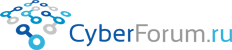С++ для начинающих
 Войти Регистрация Восстановить пароль
 Правила Карта Блоги Социальные группы Поиск Сообщения за день
 Рейтинг 4.64/11: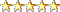0 / 0 / 0
Регистрация: 29.09.2012
Сообщений: 8
1

# LZSS принцип работы алгоритма

24.04.2013, 22:22. Просмотров 2080. Ответов 6
Метки нет(Все метки)

Приветствую! Обращаюсь сюда за помощью, так как ни как не могу разобраться с алгоритмом LZSS. После непродолжительных поисков нашел вот такой вот код, но, как я правильно понимаю, для работы шифровальщика нужен словарь. Отсюда возникает вопрос. Как его задать?
C++
 ```1 2 3 4 5 6 7 8 9 10 11 12 13 14 15 16 17 18 19 20 21 22 23 24 25 26 27 28 29 30 31 32 33 34 35 36 37 38 39 40 41 42 43 44 45 46 47 48 49 50 51 52 53 54 55 56 57 58 59 60 61 62 63 64 65 66 67 68 69 70 71 72 73 74 75 76 77 78 79 80 81 82 83 84 85 86 87 88 89 90 91 92 93 94 95 96 97 98 99 100 101 102 103 104 105 106 107 108 109 110 111 112 113 114 115 116 117 118 119 120 121 122 123 124 125 126 127 128 129 130 131 132 133 134 135 136 137 138 139 140 141 142 143 144 145 146 147 148 149 150 151 152 153 154 155 156 157 158 159 160 161 162 163 164 165 166 167 168 169 170 171 172 173 174 175 176 177 178 179 180 181 182 183 184 185 186 187 188 189 190 191 192 193 194 195 196 197 198 199 200 201 202 203 204 205 206 207 208 209 210 211 212 213 214 215 216 217 218 ``` ```#include #include #include #include   #define N                 4096        /* size of ring buffer */ #define F                   18        /* upper limit for match_length */ #define THRESHOLD        2   /* encode string into position and length                                                    if match_length is greater than this */ #define NIL                        N        /* index for root of binary search trees */   unsigned long int                 textsize = 0,        /* text size counter */                 codesize = 0,        /* code size counter */                 printcount = 0;        /* counter for reporting progress every 1K bytes */ unsigned char                 text_buf[N + F - 1];        /* ring buffer of size N,                         with extra F-1 bytes to facilitate string comparison */ int                match_position, match_length,  /* of longest match.  These are                         set by the InsertNode() procedure. */                 lson[N + 1], rson[N + 257], dad[N + 1];  /* left & right children &                         parents -- These constitute binary search trees. */ FILE        *infile, *outfile;  /* input & output files */   void InitTree(void)  /* initialize trees */ {         int  i;           /* For i = 0 to N - 1, rson[i] and lson[i] will be the right and            left children of node i.  These nodes need not be initialized.            Also, dad[i] is the parent of node i.  These are initialized to            NIL (= N), which stands for 'not used.'            For i = 0 to 255, rson[N + i + 1] is the root of the tree            for strings that begin with character i.  These are initialized            to NIL.  Note there are 256 trees. */           for (i = N + 1; i <= N + 256; i++) rson[i] = NIL;         for (i = 0; i < N; i++) dad[i] = NIL; }   void InsertNode(int r)         /* Inserts string of length F, text_buf[r..r+F-1], into one of the            trees (text_buf[r]'th tree) and returns the longest-match position            and length via the global variables match_position and match_length.            If match_length = F, then removes the old node in favor of the new            one, because the old one will be deleted sooner.            Note r plays double role, as tree node and position in buffer. */ {         int  i, p, cmp;         unsigned char  *key;           cmp = 1;  key = &text_buf[r];  p = N + 1 + key;         rson[r] = lson[r] = NIL;  match_length = 0;         for ( ; ; ) {                 if (cmp >= 0) {                         if (rson[p] != NIL) p = rson[p];                         else {  rson[p] = r;  dad[r] = p;  return;  }                 } else {                         if (lson[p] != NIL) p = lson[p];                         else {  lson[p] = r;  dad[r] = p;  return;  }                 }                 for (i = 1; i < F; i++)                         if ((cmp = key[i] - text_buf[p + i]) != 0)  break;                 if (i > match_length) {                         match_position = p;                         if ((match_length = i) >= F)  break;                 }         }         dad[r] = dad[p];  lson[r] = lson[p];  rson[r] = rson[p];         dad[lson[p]] = r;  dad[rson[p]] = r;         if (rson[dad[p]] == p) rson[dad[p]] = r;         else                   lson[dad[p]] = r;         dad[p] = NIL;  /* remove p */ }   void DeleteNode(int p)  /* deletes node p from tree */ {         int  q;                 if (dad[p] == NIL) return;  /* not in tree */         if (rson[p] == NIL) q = lson[p];         else if (lson[p] == NIL) q = rson[p];         else {                 q = lson[p];                 if (rson[q] != NIL) {                         do {  q = rson[q];  } while (rson[q] != NIL);                         rson[dad[q]] = lson[q];  dad[lson[q]] = dad[q];                         lson[q] = lson[p];  dad[lson[p]] = q;                 }                 rson[q] = rson[p];  dad[rson[p]] = q;         }         dad[q] = dad[p];         if (rson[dad[p]] == p) rson[dad[p]] = q;  else lson[dad[p]] = q;         dad[p] = NIL; }   void Encode(void) {         int  i, c, len, r, s, last_match_length, code_buf_ptr;         unsigned char  code_buf, mask;                 InitTree();  /* initialize trees */         code_buf = 0;  /* code_buf[1..16] saves eight units of code, and                 code_buf works as eight flags, "1" representing that the unit                 is an unencoded letter (1 byte), "0" a position-and-length pair                 (2 bytes).  Thus, eight units require at most 16 bytes of code. */         code_buf_ptr = mask = 1;         s = 0;  r = N - F;         for (i = s; i < r; i++) text_buf[i] = ' ';  /* Clear the buffer with                 any character that will appear often. */         for (len = 0; len < F && (c = getc(infile)) != EOF; len++)                 text_buf[r + len] = c;  /* Read F bytes into the last F bytes of                         the buffer */         if ((textsize = len) == 0) return;  /* text of size zero */         for (i = 1; i <= F; i++) InsertNode(r - i);  /* Insert the F strings,                 each of which begins with one or more 'space' characters.  Note                 the order in which these strings are inserted.  This way,                 degenerate trees will be less likely to occur. */         InsertNode(r);  /* Finally, insert the whole string just read.  The                 global variables match_length and match_position are set. */         do {                 if (match_length > len) match_length = len;  /* match_length                         may be spuriously long near the end of text. */                 if (match_length <= THRESHOLD) {                         match_length = 1;  /* Not long enough match.  Send one byte. */                         code_buf |= mask;  /* 'send one byte' flag */                         code_buf[code_buf_ptr++] = text_buf[r];  /* Send uncoded. */                 } else {                         code_buf[code_buf_ptr++] = (unsigned char) match_position;                         code_buf[code_buf_ptr++] = (unsigned char)                                 (((match_position >> 4) & 0xf0)                           | (match_length - (THRESHOLD + 1)));  /* Send position and                                         length pair. Note match_length > THRESHOLD. */                 }                 if ((mask <<= 1) == 0) {  /* Shift mask left one bit. */                         for (i = 0; i < code_buf_ptr; i++)  /* Send at most 8 units of */                                 putc(code_buf[i], outfile);     /* code together */                         codesize += code_buf_ptr;                         code_buf = 0;  code_buf_ptr = mask = 1;                 }                 last_match_length = match_length;                 for (i = 0; i < last_match_length &&                                 (c = getc(infile)) != EOF; i++) {                         DeleteNode(s);                /* Delete old strings and */                         text_buf[s] = c;        /* read new bytes */                         if (s < F - 1) text_buf[s + N] = c;  /* If the position is                                 near the end of buffer, extend the buffer to make                                 string comparison easier. */                         s = (s + 1) & (N - 1);  r = (r + 1) & (N - 1);                                 /* Since this is a ring buffer, increment the position                                    modulo N. */                         InsertNode(r);        /* Register the string in text_buf[r..r+F-1] */                 }                 if ((textsize += i) > printcount) {                         printf("%12ld\r", textsize);  printcount += 1024;                                 /* Reports progress each time the textsize exceeds                                    multiples of 1024. */                 }                 while (i++ < last_match_length) {        /* After the end of text, */                         DeleteNode(s);                                        /* no need to read, but */                         s = (s + 1) & (N - 1);  r = (r + 1) & (N - 1);                         if (--len) InsertNode(r);                /* buffer may not be empty. */                 }         } while (len > 0);        /* until length of string to be processed is zero */         if (code_buf_ptr > 1) {                /* Send remaining code. */                 for (i = 0; i < code_buf_ptr; i++) putc(code_buf[i], outfile);                 codesize += code_buf_ptr;         }         printf("In : %ld bytes\n", textsize);        /* Encoding is done. */         printf("Out: %ld bytes\n", codesize);         printf("Out/In: %.3f\n", (double)codesize / textsize); }   void Decode(void)        /* Just the reverse of Encode(). */ {         int  i, j, k, r, c;         unsigned int  flags;                 for (i = 0; i < N - F; i++) text_buf[i] = ' ';         r = N - F;  flags = 0;         for ( ; ; ) {                 if (((flags >>= 1) & 256) == 0) {                         if ((c = getc(infile)) == EOF) break;                         flags = c | 0xff00;                /* uses higher byte cleverly */                 }                                                        /* to count eight */                 if (flags & 1) {                         if ((c = getc(infile)) == EOF) break;                         putc(c, outfile);  text_buf[r++] = c;  r &= (N - 1);                 } else {                         if ((i = getc(infile)) == EOF) break;                         if ((j = getc(infile)) == EOF) break;                         i |= ((j & 0xf0) << 4);  j = (j & 0x0f) + THRESHOLD;                         for (k = 0; k <= j; k++) {                                 c = text_buf[(i + k) & (N - 1)];                                 putc(c, outfile);  text_buf[r++] = c;  r &= (N - 1);                         }                 }         } }   int main(int argc, char *argv[]) {         char  *s;                 if (argc != 4) {                 printf("'lzss e file1 file2' encodes file1 into file2.\n"                            "'lzss d file2 file1' decodes file2 into file1.\n");                 return 1;         }         if ((s = argv, s || strpbrk(s, "DEde") == NULL)          || (s = argv, (infile  = fopen(s, "rb")) == NULL)          || (s = argv, (outfile = fopen(s, "wb")) == NULL)) {                 printf("%s\n", s);  return 1;         }         if (toupper(*argv) == 'E') Encode();  else Decode();         fclose(infile);  fclose(outfile);         return 0; }```
Добавлено через 26 минут
Сформулирую по другому. Где должен находиться файл на вход и выход? В буфере?
0
 Programming Эксперт 94731 / 64177 / 26122 Регистрация: 12.04.2006 Сообщений: 116,782 24.04.2013, 22:22 Ответы с готовыми решениями: Принцип работы switchВсем доброго времени суток. Изучаю самостоятельно С++. Возник вопрос по поводу функции switch....Принцип работы программыСмысл программы: подсчет количества слов и предложений из подключенного файла. Код есть, только не...Принцип работы fstreamДопустим у нас есть файл из четырех чисел, например: 453 32 43 54. Я создаю экземпляр класса...объсните принцип работыОбъясните кому не сложно принцип работы данной программы Планируется переписывание на паскале... 6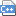4958 / 3064 / 456 Регистрация: 10.11.2010 Сообщений: 11,152 Записей в блоге: 10 24.04.2013, 22:24 2 В файловой системе. 0
 0 / 0 / 0 Регистрация: 29.09.2012 Сообщений: 8 27.04.2013, 21:39  [ТС] 3 На другом форуме (мигрирую опять сюда) ответили, что нужно задать для .exe параметры: (e file1 file2) - упаковка, (d file1 file2) - распаковка. file1 и file2 - входные и выходные данные соответственно. Задал, создал файлы в двоичном формате (.bin), но шифровальщик опять ни как не оперирует данными. Я понимаю, что я безнадежен и меня надо гнать в шею, но действительно что-то не могу разобраться... 04958 / 3064 / 456 Регистрация: 10.11.2010 Сообщений: 11,152 Записей в блоге: 10 27.04.2013, 21:44 4 Что значит: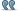Сообщение от lycanthropy999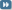но шифровальщик опять ни как не оперирует данными ? Ты хочешь чтобы он еще что-то для тебя сделал? 0
 0 / 0 / 0 Регистрация: 29.09.2012 Сообщений: 8 27.04.2013, 23:22  [ТС] 5 LZSS - это метод шифрования (сжатия и распаковки) и как от метода шифрования, я жду того, чего от него и требуется - шифрования. Но я тут явно что-то упускаю: файлы создал, параметры программы указал, но прога выдает мне синтаксическую ошибку. Видно, я неправильно задаю входные данные. Но, вот, только. Как правильно? Может еще какую-нибудь подсказочку? Я же не требую выложить все на блюдечке с золотой каемочкой. 04958 / 3064 / 456 Регистрация: 10.11.2010 Сообщений: 11,152 Записей в блоге: 10 28.04.2013, 00:00 6 Погоди.. у тебя при запуске ошибку выдает или где? Какую ошибку? Я успешно скомпилировал у себя этот код без изменений, запустил, запаковал файл, распаковал файл. Все работает. Запаковывал так: main.exe e исходный_файл запакованный_файл (e - латинская, имена файлов свои) Распаковывал так: main.exe d запакованный_файл исходный_файл (тут d - тоже латинская) Ну и разумеется между каждым параметром пробел. 2
 0 / 0 / 0 Регистрация: 29.09.2012 Сообщений: 8 28.04.2013, 00:39  [ТС] 7 Все разобрался - не указывал формат исходного и конечного файла в параметрах. Глупо... Вам, lazybiz, выражаю благодарность за помощь. 0
IT_Exp
Эксперт
87844 / 49110 / 22898
Регистрация: 17.06.2006
Сообщений: 92,604
28.04.2013, 00:39

Заказываю контрольные, курсовые, дипломные и любые другие студенческие работы здесь.

Принцип работы программ
Изучаю с++. Сейчас знаю до классов и все. И у меня возник вопрос. Получается весь принцип работы...

Getline принцип работы
Не совсем понимаю как влияет цикл. Да, выводится все сообщение, каждая срока с новой строки. Но,...

 Powered by vBulletin® Version 3.8.9Copyright ©2000 - 2020, vBulletin Solutions, Inc.